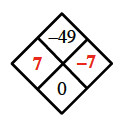### Home > MC1 > Chapter 5 > Lesson 5.2.4 > Problem5-82

5-82.

Copy and complete each of the Diamond Problems below. The pattern used in the Diamond Problems is shown at right.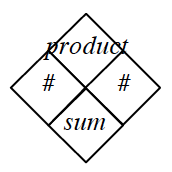1.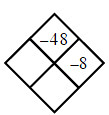Refer to problem 5-21 if you are having trouble starting this problem.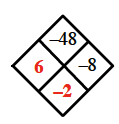1.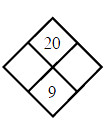Which numbers add to $9$ and multiply to $20$?

1.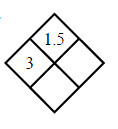What number do you need to multiply $3$ by to get $1.5$? Add this to $3$ to get the sum.

1.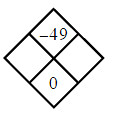The two numbers will be the same distance from $0$ on the number line, but one will be negative.